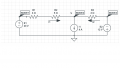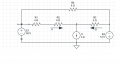# Determining the Current using mesh/nodal analysis.

Thread Starter

#### Mahmoud Salem

Joined May 6, 2017
6
Hello,
I was asked to find current I1 and I2 in the problem below using nodal analysis.I found out the current to be V=13.33V, I1=3.33A and I2=0.7278A. I was later asked to find the same currents (I1 and I2) after the addition of a new resistor using mesh analysis.After applying mesh analysis(supermesh),I got the same value for the two currents. How is it possible that the 2 currents were not altered by the new resistor? Also, How do i make sure the values I got are correct?

#### WBahn

Joined Mar 31, 2012
26,398
You make sure the values you got are correct seeing if the are consistent with the rest of the circuit.

You don't say where this "V" is that is supposedly 13.33 V. I have to assume it is probably V2, but don't make your readers assume.

Assuming that V2 = 13.33 V, if V1 = 20 V, what does that make I1? Is it 3.33 A? Seems more like 0.67 A to me.

Assuming that V2 = 13.33 V, if V3 = -12 V, what does that make I2? Is it 0.7278 A? Seems more like -12.67 A to me.

Assuming I1 and I2 are what you claim, is that consistent with I1 + I2 = 4 A? They seem to add up to 4.0578 A, which is a bit too far off for roundoff error.

So you have LOTS of red flag warnings that your answers are not correct.

Since you don't show your work, I can't begin to tell you where you went wrong.

As for how the currents can be the same despite the addition of the new resistor, consider this:

I have a 10 Ω resistor across a 12 V supply. What is the current in it? I then place a 1 Ω resistor in parallel with it across the supply. What is the current in the 10 Ω resistor?

Thread Starter

#### Mahmoud Salem

Joined May 6, 2017
6
You make sure the values you got are correct seeing if the are consistent with the rest of the circuit.

You don't say where this "V" is that is supposedly 13.33 V. I have to assume it is probably V2, but don't make your readers assume.

Assuming that V2 = 13.33 V, if V1 = 20 V, what does that make I1? Is it 3.33 A? Seems more like 0.67 A to me.

Assuming that V2 = 13.33 V, if V3 = -12 V, what does that make I2? Is it 0.7278 A? Seems more like -12.67 A to me.

Assuming I1 and I2 are what you claim, is that consistent with I1 + I2 = 4 A? They seem to add up to 4.0578 A, which is a bit too far off for roundoff error.

So you have LOTS of red flag warnings that your answers are not correct.

Since you don't show your work, I can't begin to tell you where you went wrong.

As for how the currents can be the same despite the addition of the new resistor, consider this:

I have a 10 Ω resistor across a 12 V supply. What is the current in it? I then place a 1 Ω resistor in parallel with it across the supply. What is the current in the 10 Ω resistor?
After re-checking my work,Turns out the voltage was -13.33V (V2). Sorry about that.
I would show you my work but i've done it on a scribble notebook and it seems very messy so i doubt it will be of any help to you.

#### WBahn

Joined Mar 31, 2012
26,398
After re-checking my work,Turns out the voltage was -13.33V (V2). Sorry about that.
I would show you my work but i've done it on a scribble notebook and it seems very messy so i doubt it will be of any help to you.
I figured you were off by a minus sign -- which just goes to show the value of checking your work. You don't even have to run any numbers to catch a mistake like this. Just ask what direction the two currents have to be given the proposed answer.

Even after correcting that there is still the other mismatch. If you have -13.33 V on one side of a 2 Ω resistor and -12 V on the other, is the current 0.7278 A?

Go back and check your work and figure out why you got an answer for I2 that is nearly 10% too high.

For most circuit analysis work, your answers should be correct to within a small fraction of a percent (i.e., three sig figs in the final result). The error here is on the order of 50 to 100 times what you should be willing to accept.

Thread Starter

#### Mahmoud Salem

Joined May 6, 2017
6
I figured you were off by a minus sign -- which just goes to show the value of checking your work. You don't even have to run any numbers to catch a mistake like this. Just ask what direction the two currents have to be given the proposed answer.

Even after correcting that there is still the other mismatch. If you have -13.33 V on one side of a 2 Ω resistor and -12 V on the other, is the current 0.7278 A?

Go back and check your work and figure out why you got an answer for I2 that is nearly 10% too high.

For most circuit analysis work, your answers should be correct to within a small fraction of a percent (i.e., three sig figs in the final result). The error here is on the order of 50 to 100 times what you should be willing to accept.
Yeh I have no idea how that number came up really. Must've done something wrong when i was calculating. I will be more careful when it comes to rounding up my answers from now on. I really appreciate all the feedback you've given me sir.